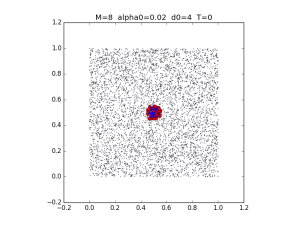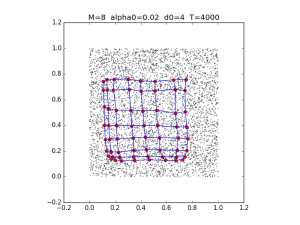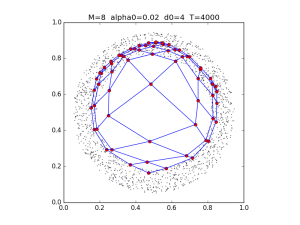# 252-ps2

Computer Science 252
Neuromorphic Computing

Assignment 2: Kohonen’s Self-Organizing Map

What I cannot create, I do not understand – Richard Feynman (1918 – 1988)

Objectives

1. Understand the Self-Organizing Map (SOM) by coding it from scratch in Python.
2. Be able to use NumPy to generate training data for SOM and other neural-net algorithms.
3. Be able to use matplotlib to display your results.

To give you something to aim for, here are two test runs of my SOM code (which was around 130 lines of Python, including comments). As you can see, I trained on two test shapes: a simple square, and more complicated ring. The square is just for initial testing; for your turnin, you only need to show one shape, as long as its nontrivial (ring, cross, etc.). The parameters I used are displayed at the top of each plot. Getting to 4000 (four thousand) iterations shouldn’t take more than a few seconds.The following steps show how I got these results. You’re free to proceed however you like, but you are likely to get your results quicker if you follow these step-by-step directions.

Part 1: Generate some training data

Create a file som.py. This file should start by importing the standard packages we’ll use throughout this course:

```import numpy as np
import matplotlib.pyplot as plt
```

Next you should aim to produce a plot like the one in the upper-left above, but without the title or the SOM network in the center (i.e., just a big square of random dots). To do this I used np.random.random, which takes a tuple specifying the size of the data you want, and returns an array of values between 0 and 1. I created a 5000×2 array this way. Then you can use plt.scatter to visualize the data. Like the plt.plot that you used in the previous assignment, this function requires a separate x and y input. So if your data is stored in array data, you can do plt.scatter(data[:,0], data[:,1], s=.2) to get a plot like the one above, where the .2 gives you tiny dots. The other trick you’ll need, to get a nice square plot, is plt.gca().set_aspect('equal').

Part 2: Create an SOM

Now it’s time to create an SOM class. The __init__ method should accept an input m specifying the size of the grid (e.g., an m of 10 will give you a 10×10 grid), and and input n specifying the dimensionality of the weights (in this case, 2, because we’re learning two-dimensional patterns). All that your __init__ needs to do is create the initial network weights u for your SOMobject. As before, np.random.random will do this for you. This time, you’ll want to pass it a three-tuple of the values (m,m,n). This will give you back an array of size m×m×n, technically called a tensor (a word that you’ll see again if you continue your study of neural networks). Now, np.random.random gives you values uniformly distributed between 0 and 1, but we want values clustered around 0.5. I was able to get these by dividing the out of np.random.random by 10 and adding 0.45.

Now that you’ve create a random SOM, you’ll want to plot it. For now, we’ll plot only the locations of the “neurons”, as red dots, rather than plotting the lines between them. To do this, you can write a nested loop that loops j over m, then k over m again, plotting u[j,k] as a red circle (input 'ro' to plt.plot). Since each u[j,k] is itself a two-dimensional value, you will treat the first value as the x coordinate, and the second as y. At this point, you may want to write a new function that takes the training data from Part 1 and the SOM from this part, and plots both the data and the SOM. That way, you can also use the function to plot the trained SOM that you’re going to get in the next step.

Part 3: Train the SOM

This is the most challenging part of the assignment – implementing the SOM algorithm from the lecture notes – which took me longer to complete than the other parts combined. To get started, add a learn method to your SOM class. This method should accept your training data from Part 1, as well as the number of iterations T, the initial learning rate α0, and the initial neighborhood distance d0. To help you with this complicated step, here are the comments from my implementation:

```
# Iterate t from 0 to T

# Compute current neighborhood radius d and learning rate alpha

# Pick an input e from the training set at random

# Find the winning unit whose weights are closest to this e

# Loop over the neighbors of this winner, adjusting their weights

```

1. If n is the number of x,y data points in your training np.random.random_integers(n-1) will give you a random index from 0 through n-1, so you can pick the input point e.
2. Finding the winner should probably be done in a separate “private” method of your SOM class. I wrote a method that accepted the randomly-chosen training point e and returned the pair of indices for the winning SOM neuron (weight) closest to e.
3. Likewise, getting the neighbors of the winner should probably be done in a distinct method. I wrote a method that accepted a pair p of indices (the winner from the previous step) and a neighborhood distance, and returned a list of pairs of indices in that neighborhood. The trick is to make sure that none of these indices is less than zero or greater than m (the size of the network).

Part 4: Plot connections between neighbors

If you did Part 3 correctly, you should see something like the upper-right figure – a distorted square grid of red points that covers most of the training data – but without the lines connecting each neuron to its neighbors. So now its time to augment your plotting code with some code to display the lines. Inside the loop that plots the red dots, add a line to plot from u[j,k] to u[j+1,k], and another to plot from u[j,k] to u[j,k+1]. This creates a classic fencepost scenario, where you have m dots and therefore need m-1 connections between them. So you’ll need to put a guard before each of these new plot statements, to make sure you don’t plot once you’ve reached the final iteration of the plotting loop.

Part 5: Use a more challenging training pattern

The square data gives a pretty nice result, but for full credit you’ll want to use a training set that has a more interesting shape, like a ring. This will require you to constrain the square output of np.random.random using logical indexing, a trick borrowed from Matlab in which you use True/False values as direct indices into an array. For example, if data contains your array of x,y training data pairs, r = (data[:,0]-.5)**2 + (data[:,1]-.5)**2 will give you the distances r of each point from the center (0.5,0.5). I’m calling these r instead of d to avoid confusion with the d variable from the SOM algorithm, but also to point out that you can treat these values as the radii of the circle on which each data point lies. Then, for example, r < 0.2 will give you the logical indices of the points lying on a disc with a radius of 0.2. To get a ring rather than a disc, you can use np.logical_and to combine this constraint with a constraint for the minimal value of r as well as the maximum 0.2. Then you can replace data with the subset of its points that satisfy these constraints. This sounds like a lot of code, but it should only take two lines!

Part 6: Polish it up

Now you can put the finishing touches on your plot: Use plt.title to add an informative title, and generally make sure that the plot comes up square and neat like the ones I’ve shown. Finally, try to factor repeated code into a function. Though getting the code to work is always the most important thing, I may check your code to see that there isn’t a lot of redundancy, and take off a few points of there is.

Extra-Credit Challenges

1. Try a shape other than ring: a cross, or an X, or some other interesting shape.
2. Make your SOM work in three dimensions; i.e., test it on a cube or sphere. This code should use the same SOM class as your two-dimensional example.
3. Show the progress of your SOM convergence in an animation.
4. Using one or more texts downloaded from the internet, replicate the results of Zhao, Li, Kohonen (2010).

What to turn in to sakai

The only file you need to turn in is your final som.py. For full credit, this script should use a nontrivial shape (ring, cross, X, whatever), first displaying the initial conditions (random network), and then the final conditions. I.e., I should see two plots like the ones in the second row of figures above (or a complete movie if you do the extra-credit option).